##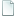Topic: Colliding a Sphere with a Plane (Read 4036 times)previous topic - next topic

0 Members and 1 Guest are viewing this topic.
• Flying Jester
•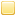• TurboSphere Developer
Colliding a Sphere with a Plane
##### May 18, 2016, 05:05:36 pm
No pun intended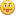I want to post how this is (easily) done, since I can't find any useful examples online. I keep needing this on and off over the years, so I might was well give it a home here.

It's actually super easy to do, but there are very few discussions of such. It's also the same as checking the distance between a point and a plane (since a sphere is just a point and a distance).

First, take a three points to define the plane and determine the normal to the plane using these points.

For reference:
Code: (javascript) [Select]
`function cross(a, b){  let x = (a.y * b.z) - (a.z * b.y);  let y = (a.z * b.x) - (a.x * b.z);  let z = (a.x * b.y) - (a.y * b.x);  return {"x":x, "y":y, "z":z};}function subtract(a, b){  return {"x":a.x - b.x, "y":a.y - b.y, "z":a.z - b.z};}function normal(a, b, c){  let da =  subtract(a, c);  let db =  subtract(b, c);  return cross(da, db);}`

Then, determine the plane's D component which is negative of the normal multiplied by one of the points.

Code: (javascript) [Select]
`function multiply(a, b){  return {"x":a.x * b.x, "y":a.y * b.y, "z":a.z * b.z};}function plane_d(normal_cmp, point){  let d_vec = subtract({"x":0, "y":0, "z":0}, multiply(normal_cmp, point));  return d_vec.x + d_vec.y + d_vec.z;}`

So, to create a plane (we will want the normal vector to be normalized for later):
Code: (javascript) [Select]
`function normalize(vec){  let m = Math.sqrt((vec.x * vec.x) + (vec.y * vec.y) + (vec.z * vec.z));  return { "x":vec.x/m, "y":vec.y/m, "z":vec.z/m };}function plane(a, b, c){  this.points = [a, b, c]; // It will be useful to know at least one point on the plane later.  this.normal = normalize(normal(a, b, c));  this.d = plane_d(this.normal, a);}`

Alright, so the very obvious part. We need to collide a line that passes through the sphere's center, from the points on the sphere's edge that are exactly the radius away in both the plane's normal and anti-normal (aka, a line going all the way through the sphere, crossing the center, and perpendicular to the plane).

Then, we generate a 3x3 matrix from this and the difference of the three points we defined the plane from (any three points on the plane would work, but here we already have some). Then, invert the matrix and multiply that by the matrix [ line.x-points.x, line.y - points.y, line.z - points.z ] (but in column order).

Code: (javascript) [Select]
`    /*     *   [ a b c ]          [ A D G ]          [  (ei-fh) -(bi-ch)  (bf-ce) ]     * P [ d e f ] = det(A) [ B E H ] = det(A) [ -(di-fg)  (ai-cg) -(af-cd) ]     *   [ g h i ]          [ C F I ]          [  (dh-eg) -(ab-bg)  (ae-bd) ]     * For the line of points A and B and the plane of points 0, 1, 2     *        [  (ei-fh) -(bi-ch)  (bf-ce) ] | [ (Xa-Xb) (X1-X0) (X2-X0) ]     * det(A) [ -(di-fg)  (ai-cg) -(af-cd) ] | [ (Ya-Yb) (Y1-Y0) (Y2-Y0) ] = W     *        [  (dh-eg) -(ab-bg)  (ae-bd) ] | [ (Za-Zb) (Z1-Z0) (Z2-Z0) ]     *     * det(A) = 1/(a * A + b * B + c * C)     * det(A) = 1/((Xa-Xb) * (ei-fh) + (X1-X0) * (ch-bi) + (X2-X0) * (bf-ce))     *                   [ Xa-X0 ]   [ T ]     * Thus, inverse W * [ Ya-Y0 ] = [ U ]     *                   [ Za-Z0 ]   [ V ]     * T is between 0 and 1 if the intersection is along the line.     * (U+V) <= 1 if the intersection is withing the three points.     */plane.prototype.whyareyoudoingthistome = function(sphere){  line = {"x1":sphere.x + this.normal.x * sphere.radius, "y1":sphere.y + this.normal.y * sphere.radius, "z1":sphere.z + this.normal.z * sphere.radius,    "x2":sphere.x - this.normal.x * sphere.radius, "y2":sphere.y - this.normal.y * sphere.radius, "z2":sphere.z - this.normal.z * sphere.radius};  let a = line.x1 - line.x2, b = this.points.x - this.points.x, c = this.points.x - this.points.x,    d = line.y1 - line.y2, e = this.points.y - this.points.y, f = this.points.y - this.points.y,    g = line.z1 - line.z2, e = this.points.z - this.points.z, f = this.points.z - this.points.z;  // The commented out portions would only be useful if we wanted to determine if the intersection was bound by the three input points.  let A = ((e*i)-(f*h)), B = ((c*h)-(b*i)), C = ((b*f)-(c*e)),    D = ((f*g)-(d*i)),/* E = ((a*i)-(c*g)), F = ((c*d)-(a*f)), */    G = ((d*h)-(e*g)) /* H = ((b*g)-(a*b)), I = ((a*e)-(b*d)) */ ;  let Q = line.x1 - this.points.x, R = line.y1 - this.points.y, S = line.z1 - this.points.z;    // Inversion coefficient, of course. Mismatched to match the final matrix rotation for inversion.  let det = 1 / ((a*A) + (b*D) + (c*G));  let T = ((A*Q)+(B*R)+(C*S)) * det;  // U and V are UV coordinates on the triangle along which we intersected. If you were ray-tracing, for instance, this would be texture coordinates relative to the intersection.  // let U = (float)((D*R)+(E*S)+(F*T)) * det_a;  // let V = (float)((G*R)+(H*S)+(I*T)) * det_a;    // T is actually the T position along the line where the plane and the line intersect.  return T >= 0 && T <= 1;}`

So obvious, so clear.

Except this is actually the really hard way. I've done it this way for some years, but today I made a discovery that made me feel like an idiot.

Here is the easy way

The D coordinate of the plane's equation, if the normal vector has been normalized before D was calculated, is the distance from the point to the plane.

Code: (javascript) [Select]
`plane.prototype.collideSphere(sphere){  let point = multiply(sphere, this.normal);  let distance1 = point.x + point.y + point.z + this.d;  return Math.abs(distance1 ) < sphere.radius;}`

...

Sometimes I feel that my calculus classes in college let me down me in some ways.Re: Colliding a Sphere with a Plane
##### Reply #1 – May 19, 2016, 11:42:48 am
During your whole derivation, you didn't mention projections once, which is the obvious tool to use to get the answer here.  In the case that the plane goes through the origin, project the sphere center onto a unit normal of the  plane, then compare the length of this vector to the spheres radius.  In the case the plane doesn't go through the origin, subtract off any vector in the plane from the sphere center and do the same process.  This should give your final result without any guesswork.

Some corrections to your code: the multiply function makes no sense.  At no point is coordinate-wise vector multiplication a good function to define in linear algebra.  What you should be defining is the dot product instead, which is what you use anyway when you do point.x+point.y+point.z.

Speaking of which, how does that final answer make any sense if this.d is defined as plane_d(this.normal,a), which returns a vector, while in the final answer is the sum of three numbers and that vector?

As homework, go to https://en.wikipedia.org/wiki/Vector_projection, read it all, and then solve the problem again using dot products and projects.  Also to increase your understanding, write a 2d version for circles and lines since the cross product is not defined in two dimensions, so you'll have to find the normal in a more general way.

• Flying Jester
•• TurboSphere Developer
Re: Colliding a Sphere with a Plane
##### Reply #2 – May 19, 2016, 12:39:15 pm
The first solution was a method using line intersection with planes. I could have used projection, but my point in the end was that it is actually much, much simpler anyway.

Speaking of which, how does that final answer make any sense if this.d is defined as plane_d(this.normal,a), which returns a vector, while in the final answer is the sum of three numbers and that vector?

The D value is the D of the plane's equation. If you normalized the normal vector before this, then put any point into the plane's equation, then result is the distance away from the plane along the normal (or more accurately, the difference from the point D along a normal vector from the origin to the point you solved for).

I should note that while translating this code to JS, I did make an error (now fixed) in the plane_d function.• Fat Cerberus
••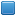• Global Moderator
• Sphere Developer
Re: Colliding a Sphere with a Plane
##### Reply #3 – May 19, 2016, 01:22:59 pm
If the Wikipedia article is right, then you don't even need to calculate the normal -- you can find the distance given just the definition of the plane and the point.  Wikipedia says that the distance D from a point P to a plane abcd is:

D = abs(a*P.x + b*P.y + c*P.z + d) / sqrt(a^2 + b^2 + c^2)

And if abc are normalized (such that sqrt(...) = 1), you can avoid the division too.  Linear algebra isn't really my strong suit, so I'm always on the lookout for simple solutions like this.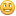Sphere 5.5.2 - neoSphere engine - Cell compiler - SSj debugger

• Flying Jester
•• TurboSphere Developer
Re: Colliding a Sphere with a Plane
##### Reply #4 – May 19, 2016, 01:47:13 pm
@Lord English: Exactly, although the wikipedia article suffers from the same issue all the articles I found do (as in it's almost totally unreadable unless you are mathematically inclined enough to basically figure it out on your own).

That's what my final function actually does. You do need the normal to determine the plane's equation, though. That's what the A, B and C are in a plane's equation (the x, y, and z of the normal vector).

Which also explains the plane's equation in a different way. The D is actually the distance of the plane as it intersects a normal vector from the origin. Which makes sense, since if you plug the origin back into the equation, you just get D...the distance between the point you plugged in and the plane.Re: Colliding a Sphere with a Plane
##### Reply #5 – May 19, 2016, 02:59:08 pm

I should note that while translating this code to JS, I did make an error (now fixed) in the plane_d function.

No, this code is still wrong.  As I said, plane_d is returning a VECTOR and it's assigned to this.d.  It doesn't make sense to add point.x+point.y+point.z+this.d.

Which also explains the plane's equation in a different way. The D is actually the distance of the plane as it intersects a normal vector from the origin. Which makes sense, since if you plug the origin back into the equation, you just get D...the distance between the point you plugged in and the plane.

Edit: I've removed this comment, it was mainly about being careful of the normal vector being a unit vector as well, which is not generally assumed for normal vectors.

Regardless, this fact for a plane equation is easily derivable from projections which are a lot less complicated than you think they are:

a, b vectors
<a,b> = a.x*b.x+a.y*b.y+a.z*b.z
|a| = root(a.x*a.x+a.y*a.y+a.z*a.z)

<a,b> = |a|*|b| cos(angle between vectors a and b)

So, the projection of a onto b, if b is a unit vector is <a,b> in the direction of b, hence <a,b> is the value of a_1 in this diagram https://upload.wikimedia.org/wikipedia/commons/thumb/9/98/Projection_and_rejection.png/200px-Projection_and_rejection.png

So, once you know that, it's a lot easier to derive the correct answer involving the dot product <a,b> (what you've written as point.x+point.y+point.z) and d.

• Fat Cerberus
••• Global Moderator
• Sphere Developer
Re: Colliding a Sphere with a Plane
##### Reply #6 – May 19, 2016, 05:41:26 pm
Uppercase D is distance (as in the distance from the point to the plane), lowercase d is the "d" in the plane equation, which is a scalar (NOT a vector).  So yeah, I think plane_d(), as posted, is incorrect.  But overall I agree that FJ's solution is the simplest way to do it, as long as you only care about spheres and planes.  I doubt it scales to more complex shapes and topologies, though.
Sphere 5.5.2 - neoSphere engine - Cell compiler - SSj debugger
•